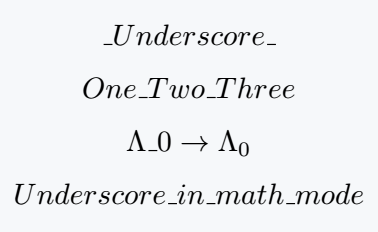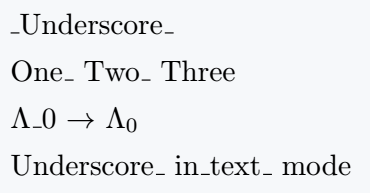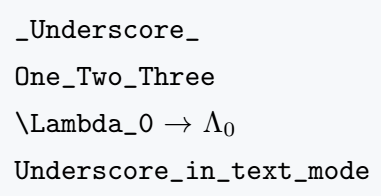# How to display underscore(m_n) in LaTeX?

In LaTeX, the underscore character is commonly used to write subscripts. So you cannot print the character directly in the document.

In this tutorial, we will discuss how we can print the underscore character in a latex document.

## Underscore in math mode

LaTeX provides a default command which is \textunderscore. This command allows you to print the underscore character in a latex document.

You can also use \_ to print this character. And better if you use like this {\_}.

\documentclass{article}
\begin{document}
$\_ Underscore \_$
$One{\_} Two{\_} Three$
$\Lambda \textunderscore 0 \rightarrow \Lambda_0$
$Underscore{\_} in \ math{\_} mode$
\end{document}

Output :## Underscore in text mode

You can use the same commands to use the underscore character in text mode. The \textunderscore and {\_} commands also work in text mode.

\documentclass{article}
\begin{document}
\textunderscore Underscore\textunderscore\\[4pt]
One{\_} Two{\_} Three\\[4pt]
$\Lambda$\textunderscore 0 $\rightarrow$ $\Lambda_0$\\[4pt]
Underscore{\_} in\textunderscore text{\_} mode
\end{document}

Output :## Another way to print underscore

LaTeX has a default command which is \verb|..|. The function of this command is to print any character or command directly to a latex document without compiling.

You just need to put the character or command in it |..|. But in this case, the font style of the output will be slightly different.

And it works in both text mode and math mode. take a look

\documentclass{article}
\begin{document}
\verb|_Underscore_|\\[4pt]
\verb|One_Two_Three|\\[4pt]
\verb|\Lambda_0| $\rightarrow$ $\Lambda_0$\\[4pt]
\verb|Underscore_in_text_mode|\\[4pt]
\end{document}

Output :#### Md Jidan Mondal

LaTeX expert with over 10 years of experience in document preparation and typesetting. Specializes in creating professional documents, reports, and presentations using LaTeX.